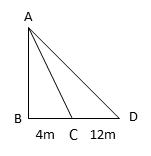# CBSE 2017 Board Paper

10th Standard Math - Set 3 - Q11. Trigonometry

#### Video ExplanationLet AB be the tower. Let AB = h
Points C and D are 4m and 16m from B

Let the angle of elevation from C, ∠BCA = θ
Because the angles of elevation from C and D are complementary, the angle of elevation from point D, ∠BDA = 90 – θ

tan θ = $$frac{\text{opposite side}}{\text{adjacent side}}$ = $\frac{AB}{BC}$ = $\frac{h}{4}$ Similarly, tan$90 – θ) = $$frac{AB}{BD}$ = $\frac{h}{16}$ .......$1)

But, tan (90 – θ) = cot θ
cot θ = $$frac{\text{adjacent side}}{\text{opposite side}}$ = $\frac{BC}{AB}$ = $\frac{4}{h}$ .......$2)

Equating tan (90 – θ) = $\frac{h}{16}$ to cot θ = $\frac{4}{h}$ we get $\frac{h}{16}$ = $\frac{4}{h}$
or h2 = 64
or h = 8.
The height of the tower is 8m.

.